## 1. IntroductionThe metrica package was developed to visualize and compute the level of agreement between observed ground-truth values and model-derived (e.g., mechanistic or empirical) predicted.

This package is intended to fit into the following workflow:

1. a data set containing the observed values is used to train a model
2. the trained model is used to generate predicted
3. a data frame containing at least the observed and model-predicted values is created
4. metrica package is used to compute and evaluate the classification model based on observed and predicted values
5. metrica package is used to visualize model fit and selected fit metrics

This vignette introduces the functionality of the metrica package applied to observed and model-predicted values of a binary land cover classification scenario, where the two classes are vegetation (1) and non-vegetation (0)).

## Libraries

library(metrica)
library(dplyr)
library(purrr)
library(tidyr)

## 2. Example datasets

### 2.1. Kansas Land Cover dataFigure 1. This binary classification dataset corresponds to a Random Forest model using a 70:30 training:testing split to predict vegetation vs. other land coverage. This exercise focused on pixel level classification. The image is showing the classification map where yellow areas are associated to non-vegetation pixels (other), and the green areas to those classified as vegetation.

Now we load the binary land_cover data set already included with the metrica package. This data set contains two columns:

• predicted: model-predicted (random forest) land cover, being vegetation = 1 and other = 0,

• actual: ground-truth observed land cover, being 0 = vegetation and 1 = other

# Load
binary_landCover <- metrica::land_cover

# Printing first observations
#>   actual predicted
#> 1      0         0
#> 2      1         1
#> 3      1         1
#> 4      0         0
#> 5      0         0
#> 6      1         1

### 2.2. Maize PhenologyFigure 2. This multiclass classification dataset corresponds to a Random Forest model using a 70:30 training:testing split to predict maize vegetation vs. other land coverage. This exercise focused on field level classification. The image is showing, in dark grey shapes, the fields used as the ground-truth locations to develop the model.

Now we load the multinomial maize_phenology data set, which is also already included with the metrica package. This multiclass data set presents 16 different classes corresponding to phenological stages of the maize (Zea Mays (L.)) crop.

# Load
multi_maize_phen <- metrica::maize_phenology

# Printing first observations
#>   actual predicted
#> 1     R1       V18
#> 2     R2        R1
#> 3     R2        R1
#> 4     R2        R2
#> 5     R2        R2
#> 6     R2        R2

## 3 Visual assessment of agreement

### 3.1 Confusion matrix

The simplest way to visually assess agreement between observed and predicted classes is with a confusion matrix.

We can use the function confusion_matrix() from the metrica package.

The function requires specifying:

• the data frame object name (data argument)

• the name of the column containing observed values (obs argument)

• the name of the column containing predicted values (pred argument)

The output of the confusion_matrix() function is either a table (plot = FALSE) or a ggplot2 object (plot = TRUE) that can be further customized:

### 3.1. Binary

# a. Print
binary_landCover %>% confusion_matrix(obs = actual, pred = predicted,
plot = FALSE,
unit = "count")
#>          OBSERVED
#> PREDICTED   0   1
#>         0 181   6
#>         1   6  92

# b. Plot
binary_landCover %>% confusion_matrix(obs = actual, pred = predicted,
plot = TRUE,
colors = c(low="#ffe8d6" , high="#892b64"),
unit = "count")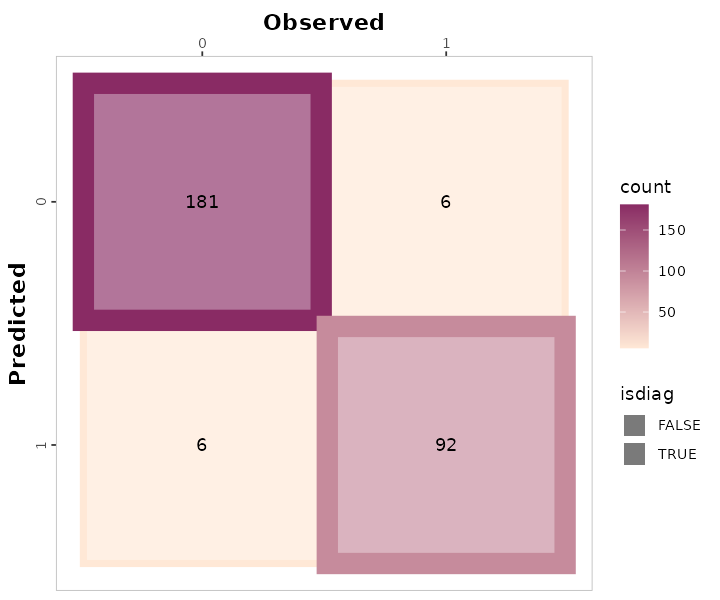# c. Unit = proportion
binary_landCover %>% confusion_matrix(obs = actual, pred = predicted,
plot = TRUE,
colors = c(low="#f9dbbd" , high="#892b64"),
unit = "proportion")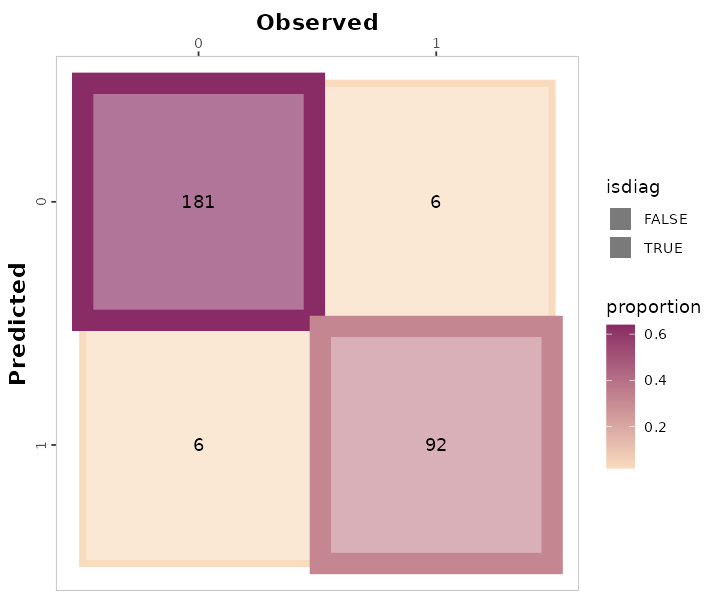### 3.2. Multiclass

# a. Print
multi_maize_phen %>% confusion_matrix(obs = actual, pred = predicted,
plot = FALSE,
unit = "count")
#>          OBSERVED
#> PREDICTED R1 R2 R3 R4 R5 R6 V10 V12 V13 V14 V15 V16 V18 V19 V9 VT
#>       R1   0  2  0  0  0  0   0   0   0   0   0   0   0   0  0  0
#>       R2   0  4  0  0  0  0   0   0   0   1   0   0   0   0  0  0
#>       R3   0  1  2  0  0  0   0   0   0   0   0   0   0   0  0  0
#>       R4   0  0  0  6  0  0   0   0   0   0   0   0   0   0  0  0
#>       R5   0  0  0  0 12  1   0   0   0   0   0   0   0   0  0  0
#>       R6   0  0  0  0  3 32   0   0   0   0   0   0   0   0  0  0
#>       V10  0  0  0  0  0  0   6   0   0   1   0   0   0   0  0  0
#>       V12  0  0  0  0  0  0   0   7   1   0   0   0   0   0  0  0
#>       V13  0  0  0  0  0  0   0   0   6   0   0   0   0   0  0  0
#>       V14  0  0  0  0  0  0   0   0   0   2   0   0   0   0  0  0
#>       V15  0  0  0  0  0  0   0   0   0   0   1   0   0   0  0  1
#>       V16  0  0  0  0  0  0   0   0   0   0   0   2   0   0  0  0
#>       V18  1  0  0  0  0  0   0   0   0   0   0   0   4   0  0  0
#>       V19  0  0  0  0  0  0   0   0   0   0   0   0   0   2  0  0
#>       V9   0  0  0  0  0  0   0   0   0   0   0   0   0   0  2  0
#>       VT   0  0  0  0  0  0   0   0   0   0   0   0   0   0  0  3

# b. Plot
multi_maize_phen %>% confusion_matrix(obs = actual, pred = predicted,
plot = TRUE,
colors = c(low="grey85" , high="steelblue"),
unit = "count")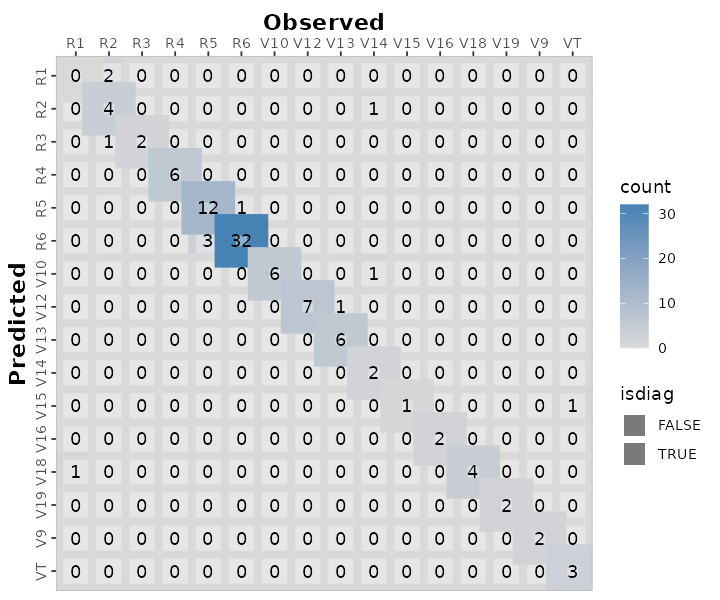## 4. Numerical assessment of agreement

The metrica package contains functions for 26 scoring rules to assess the agreement between observed and predicted values for classification data.

A list with all the the classification metrics including their name, definition, details, formula, and function name, please check here.

All of the metric functions take at least three arguments:

• the data frame object name (data argument, optional)

• the name of the column containing observed values (obs argument)

• the name of the column containing predicted values (pred argument)

• an integer (1 or 2) indicating the alphanumerical order of the positive event (pos_level argument, Default = 2)

• a TRUE/FALSE indicating to estimate metrics for each single class (atom argument, Default = FALSE). This argument is only functional for multiclass datasets.

• a TRUE/FALSE indicating to store the numeric result as a list (tidy argument, Default = FALSE), or as a data frame (tidy = TRUE).

### 4.1. Single metrics

The user can choose to calculate a single metric, or to calculate all metrics at once.

To calculate a single metric, the metric function can be called. For example, to calculate $$accuracy$$, we can use the accuracy() function:

# Binary
binary_landCover %>% accuracy(data = ., obs = actual, pred = predicted, tidy = TRUE)
#>    accuracy
#> 1 0.9578947

# Multiclass
maize_phenology %>% accuracy(data = ., obs = actual, pred = predicted, tidy = TRUE)
#>    accuracy
#> 1 0.8834951

Or considering imbalanced observations across classes we can call the balacc() function for balanced accuracy:

# Binary
binary_landCover %>% balacc(data = ., obs = actual, pred = predicted, tidy = TRUE)
#>     balacc
#> 1 0.953345

# Multiclass
maize_phenology %>% balacc(data = ., obs = actual, pred = predicted, tidy = TRUE)
#>      balacc
#> 1 0.9160466

Similarly, to calculate precision, we can use the precision() function:

# Binary
binary_landCover %>% precision(data = ., obs = actual, pred = predicted, tidy = TRUE)
#>   precision
#> 1 0.9387755

# Multiclass
maize_phenology %>% precision(data = ., obs = actual, pred = predicted, tidy = TRUE)
#>   precision
#> 1 0.8335108

### 4.2. Metrics summary

The user can also calculate all metrics at once using the function metrics_summary():


# Get all at once with metrics_summary()
# Binary
binary_landCover %>% metrics_summary(data = ., obs = actual, pred = predicted, type = "classification")
#>         Metric        Score
#> 1     accuracy   0.95789474
#> 2   error_rate   0.04210526
#> 3    precision   0.93877551
#> 4       recall   0.93877551
#> 5  specificity   0.96791444
#> 6       balacc   0.95334497
#> 7       fscore   0.93877551
#> 8          agf   0.93877551
#> 9        gmean   0.95323364
#> 10        khat   0.90668995
#> 11         mcc   0.90668995
#> 12         fmi   0.93877551
#> 13         bmi   0.90668995
#> 14         csi   0.32974910
#> 15      deltap   0.90668995
#> 16       posLr  29.25850340
#> 17       negLr   0.06325403
#> 18         dor 462.55555556
#> 19         npv   0.96791444
#> 20         FPR   0.03208556
#> 21         FNR   0.06122449
#> 22         FDR   0.06122449
#> 23         FOR   0.03208556
#> 24      preval   0.34385965
#> 25    preval_t   0.50382084
#> 26     AUC_roc   0.41849817

# Multiclass
multi_maize_phen %>% metrics_summary(data = ., obs = actual, pred = predicted, type = "classification")
#> Warning in metrica::fscore(data = ~., obs = ~actual, pred = ~predicted, : For
#> multiclass cases, the fscore should be estimated at a class level. Please,
#> consider using atom = TRUE
#> Warning in metrica::agf(data = ~., obs = ~actual, pred = ~predicted, pos_level
#> = pos_level): For multiclass cases, the agf should be estimated at a class
#> level. Please, consider using atom = TRUE
#> Warning in metrica::fmi(data = ~., obs = ~actual, pred = ~predicted, pos_level
#> = pos_level): The Fowlkes-Mallows Index is not available for multiclass cases.
#> The result has been recorded as NaN.
#> Warning in metrica::preval(data = ~., obs = ~actual, pred = ~predicted, : For
#> multiclass cases, prevalence should be estimated at a class level. A NaN has
#> been recorded as the result. Please, use atom = TRUE
#> Warning in metrica::preval_t(data = ~., obs = ~actual, pred = ~predicted, : For multiclass cases, prevalence threshold should be estimated at a class level.
#>       A NaN has been recorded as the result. Please, use atom = TRUE.
#>         Metric        Score
#> 1     accuracy 8.834951e-01
#> 2   error_rate 1.165049e-01
#> 3    precision 8.335108e-01
#> 4       recall 8.405168e-01
#> 5  specificity 9.915764e-01
#> 6       balacc 9.160466e-01
#> 7       fscore 8.369991e-01
#> 8          agf 8.370017e-01
#> 9        gmean 9.129275e-01
#> 10        khat 8.624527e-01
#> 11         mcc 8.636129e-01
#> 12         fmi          NaN
#> 13         bmi 8.320932e-01
#> 14         csi 5.577765e-02
#> 15      deltap 8.254693e-01
#> 16       posLr 9.978151e+01
#> 17       negLr 1.608381e-01
#> 18         dor 6.203850e+02
#> 19         npv 9.919585e-01
#> 20         FPR 8.423572e-03
#> 21         FNR 1.594832e-01
#> 22         FDR 1.664892e-01
#> 23         FOR 8.041482e-03
#> 24      preval          NaN
#> 25    preval_t          NaN
#> 26     AUC_roc 9.160466e-01

Alternatively, if the user is only looking for specific metrics, within the same function metrics_summary(), the user can pass a list of desired metrics using the argument “metrics_list” as follows:

# Get a selected list at once with metrics_summary()
selected_class_metrics <- c("accuracy", "precision", "recall", "fscore")

# Binary
bin_sum <- binary_landCover %>%
metrics_summary(data = .,
obs = actual, pred = predicted,
type = "classification",
metrics_list = selected_class_metrics, pos_level = 1)

# Multiclass
multi_maize_phen %>%
metrics_summary(data = .,
obs = actual, pred = predicted,
type = "classification",
metrics_list = selected_class_metrics)
#> Warning in metrica::fscore(data = ~., obs = ~actual, pred = ~predicted, : For
#> multiclass cases, the fscore should be estimated at a class level. Please,
#> consider using atom = TRUE
#> Warning in metrica::agf(data = ~., obs = ~actual, pred = ~predicted, pos_level
#> = pos_level): For multiclass cases, the agf should be estimated at a class
#> level. Please, consider using atom = TRUE
#> Warning in metrica::fmi(data = ~., obs = ~actual, pred = ~predicted, pos_level
#> = pos_level): The Fowlkes-Mallows Index is not available for multiclass cases.
#> The result has been recorded as NaN.
#> Warning in metrica::preval(data = ~., obs = ~actual, pred = ~predicted, : For
#> multiclass cases, prevalence should be estimated at a class level. A NaN has
#> been recorded as the result. Please, use atom = TRUE
#> Warning in metrica::preval_t(data = ~., obs = ~actual, pred = ~predicted, : For multiclass cases, prevalence threshold should be estimated at a class level.
#>       A NaN has been recorded as the result. Please, use atom = TRUE.
#>      Metric     Score
#> 1  accuracy 0.8834951
#> 2 precision 0.8335108
#> 3    recall 0.8405168
#> 4    fscore 0.8369991

### 4.3. Class wise metrics (atom = TRUE)

For multiclass cases, most of the classification metrics can (and should) be estimated at the class level and not simply as an overall average across classes. With the exceptions of kappa (khat), mcc, fmi, and AUC_roc, all classification metrics can be estimated at the class level using the argument atom = TRUE, as follows:

# Precision
maize_phenology %>% metrica::precision(obs = actual, pred = predicted, atom = TRUE, tidy = TRUE)
#>     precision
#> R1  0.0000000
#> R2  0.8000000
#> R3  0.6666667
#> R4  1.0000000
#> R5  0.9230769
#> R6  0.9142857
#> V10 0.8571429
#> V12 0.8750000
#> V13 1.0000000
#> V14 1.0000000
#> V15 0.5000000
#> V16 1.0000000
#> V18 0.8000000
#> V19 1.0000000
#> V9  1.0000000
#> VT  1.0000000
# Recall
maize_phenology %>% metrica::recall(obs = actual, pred = predicted, atom = TRUE, tidy = TRUE)
#>        recall
#> R1  0.0000000
#> R2  0.5714286
#> R3  1.0000000
#> R4  1.0000000
#> R5  0.8000000
#> R6  0.9696970
#> V10 1.0000000
#> V12 1.0000000
#> V13 0.8571429
#> V14 0.5000000
#> V15 1.0000000
#> V16 1.0000000
#> V18 1.0000000
#> V19 1.0000000
#> V9  1.0000000
#> VT  0.7500000
# Specificity
maize_phenology %>% metrica::specificity(obs = actual, pred = predicted, atom = TRUE, tidy = TRUE)
#>          spec
#> R1  0.9803922
#> R2  0.9895833
#> R3  0.9900990
#> R4  1.0000000
#> R5  0.9886364
#> R6  0.9571429
#> V10 0.9896907
#> V12 0.9895833
#> V13 1.0000000
#> V14 1.0000000
#> V15 0.9901961
#> V16 1.0000000
#> V18 0.9898990
#> V19 1.0000000
#> V9  1.0000000
#> VT  1.0000000

# atom = TRUE available for more functions available (remove #)
# F-score
# maize_phenology %>% metrica::fscore(obs = actual, pred = predicted, atom = TRUE, tidy = TRUE)
# maize_phenology %>% metrica::agf(obs = actual, pred = predicted, atom = TRUE, tidy = TRUE)
# # G-mean
# maize_phenology %>% metrica::gmean(obs = actual, pred = predicted, atom = TRUE, tidy = TRUE)
# # Negative predictive value
# maize_phenology %>% metrica::npv(obs = actual, pred = predicted, atom = TRUE, tidy = TRUE)
# # Prevalence
# maize_phenology %>% metrica::preval(obs = actual, pred = predicted, atom = TRUE, tidy = TRUE)
# # Prevalence threshold
# maize_phenology %>% metrica::preval_t(obs = actual, pred = predicted, atom = TRUE, tidy = TRUE)
# # False omission rate
# maize_phenology %>% metrica::FOR(obs = actual, pred = predicted, atom = TRUE, tidy = TRUE)
# # False detection rate
# maize_phenology %>% metrica::FDR(obs = actual, pred = predicted, atom = TRUE, tidy = TRUE)
# # False positive rate
# maize_phenology %>% metrica::FPR(obs = actual, pred = predicted, atom = TRUE, tidy = TRUE)
# # Falase negative rate
# maize_phenology %>% metrica::FNR(obs = actual, pred = predicted, atom = TRUE, tidy = TRUE)
# # Delta-p
# maize_phenology %>% metrica::deltap(obs = actual, pred = predicted, atom = TRUE, tidy = TRUE)
# # Critical Success Index
# maize_phenology %>% metrica::csi(obs = actual, pred = predicted, atom = TRUE, tidy = TRUE)
# # Bookmaker Informedness
# maize_phenology %>% metrica::bmi(obs = actual, pred = predicted, atom = TRUE, tidy = TRUE)
# # Positive likelihood ratio
# maize_phenology %>% metrica::posLr(obs = actual, pred = predicted, atom = TRUE, tidy = TRUE)
# # Negative likelihood ratio
# maize_phenology %>% metrica::negLr(obs = actual, pred = predicted, atom = TRUE, tidy = TRUE)
# # Diagnostic odds ratio
# maize_phenology %>% metrica::dor(obs = actual, pred = predicted, atom = TRUE, tidy = TRUE)

### 4.4. Multiple models

In some cases, multiple runs of a model are available to compare vs. observed values (e.g. cross-validation folds). Thus, we can also fit the agreement analysis for several datasets as follows:


set.seed(15)
# Let's simulated two extra runs of the same model for Land Cover
fold_2 <- data.frame(actual = sample(c(0,1), 285, replace = TRUE),
predicted = sample(c(0,1), 285, replace = TRUE))
fold_3 <- data.frame(actual = sample(c(0,1), 285, replace = TRUE),
predicted = sample(c(0,1), 285, replace = TRUE))

# a. Create nested df with the folds
binary_nested_folds <- bind_rows(list(fold_1 = binary_landCover,
fold_2 = fold_2,
fold_3 = fold_3),
.id = "id") %>%
dplyr::group_by(id) %>% tidyr::nest()

#> # A tibble: 3 × 2
#> # Groups:   id 
#>   id     data
#>   <chr>  <list>
#> 1 fold_1 <tibble [285 × 2]>
#> 2 fold_2 <tibble [285 × 2]>
#> 3 fold_3 <tibble [285 × 2]>

# b. Run
binary_folds_summary <- binary_nested_folds %>%
# Store metrics in new.column "performance"
dplyr::mutate(performance =
purrr::map(data,
~metrica::metrics_summary(data = .,
obs = actual, pred = predicted,
type = "classification"))) %>%
dplyr::select(-data) %>%
tidyr::unnest(cols = performance) %>%
dplyr::arrange(Metric)

#> # A tibble: 6 × 3
#> # Groups:   id 
#>   id     Metric   Score
#>   <chr>  <chr>    <dbl>
#> 1 fold_1 AUC_roc 0.418
#> 2 fold_2 AUC_roc 0.446
#> 3 fold_3 AUC_roc 0.513
#> 4 fold_1 FDR     0.0612
#> 5 fold_2 FDR     0.620
#> 6 fold_3 FDR     0.471

#### 4.4.1.1. Using group_map()

non_nested_folds <- binary_nested_folds %>% unnest(cols = "data")

# Using group_map()
binary_folds_summary_2 <- non_nested_folds %>%
dplyr::group_by(id) %>%
dplyr::group_map(~metrics_summary(data = ., obs = actual, pred = predicted, type = "classification"))

binary_folds_summary_2
#> []
#>         Metric        Score
#> 1     accuracy   0.95789474
#> 2   error_rate   0.04210526
#> 3    precision   0.93877551
#> 4       recall   0.93877551
#> 5  specificity   0.96791444
#> 6       balacc   0.95334497
#> 7       fscore   0.93877551
#> 8          agf   0.93877551
#> 9        gmean   0.95323364
#> 10        khat   0.90668995
#> 11         mcc   0.90668995
#> 12         fmi   0.93877551
#> 13         bmi   0.90668995
#> 14         csi   0.32974910
#> 15      deltap   0.90668995
#> 16       posLr  29.25850340
#> 17       negLr   0.06325403
#> 18         dor 462.55555556
#> 19         npv   0.96791444
#> 20         FPR   0.03208556
#> 21         FNR   0.06122449
#> 22         FDR   0.06122449
#> 23         FOR   0.03208556
#> 24      preval   0.34385965
#> 25    preval_t   0.50382084
#> 26     AUC_roc   0.41849817
#>
#> []
#>         Metric      Score
#> 1     accuracy  0.4350877
#> 2   error_rate  0.5649123
#> 3    precision  0.3795620
#> 4       recall  0.4062500
#> 5  specificity  0.4585987
#> 6       balacc  0.4324244
#> 7       fscore  0.3924528
#> 8          agf  0.3925343
#> 9        gmean  0.4316315
#> 10        khat -0.1342793
#> 11         mcc -0.1345500
#> 12         fmi  0.3926794
#> 13         bmi -0.1351513
#> 14         csi  0.2488038
#> 15      deltap -0.1339515
#> 16       posLr  0.7503676
#> 17       negLr  1.2947049
#> 18         dor  0.5795666
#> 19         npv  0.4864865
#> 20         FPR  0.5414013
#> 21         FNR  0.5937500
#> 22         FDR  0.6204380
#> 23         FOR  0.5135135
#> 24      preval  0.4491228
#> 25    preval_t  0.5151462
#> 26     AUC_roc  0.4457023
#>
#> []
#>         Metric      Score
#> 1     accuracy 0.51578947
#> 2   error_rate 0.48421053
#> 3    precision 0.52857143
#> 4       recall 0.50684932
#> 5  specificity 0.52517986
#> 6       balacc 0.51601459
#> 7       fscore 0.51748252
#> 8          agf 0.51752352
#> 9        gmean 0.51593318
#> 10        khat 0.03199606
#> 11         mcc 0.03202444
#> 12         fmi 0.51759643
#> 13         bmi 0.03202917
#> 14         csi 0.34741784
#> 15      deltap 0.03201970
#> 16       posLr 1.06745538
#> 17       negLr 0.93901295
#> 18         dor 1.13678451
#> 19         npv 0.50344828
#> 20         FPR 0.47482014
#> 21         FNR 0.49315068
#> 22         FDR 0.47142857
#> 23         FOR 0.49655172
#> 24      preval 0.51228070
#> 25    preval_t 0.50444076
#> 26     AUC_roc 0.51257025

#### 4.4.1.2. Using summarise()


# Using summarise()
binary_folds_summary_3 <- non_nested_folds %>%
dplyr::group_by(id) %>%
dplyr::summarise(metrics_summary(obs = actual, pred = predicted, type = "classification")) %>%
dplyr::arrange(Metric)
#> Warning: Returning more (or less) than 1 row per summarise() group was deprecated in
#> dplyr 1.1.0.
#> ℹ Please use reframe() instead.
#> ℹ When switching from summarise() to reframe(), remember that reframe()
#>   always returns an ungrouped data frame and adjust accordingly.
#> Call lifecycle::last_lifecycle_warnings() to see where this warning was
#> generated.
#> summarise() has grouped output by 'id'. You can override using the .groups
#> argument.

binary_folds_summary_3
#> # A tibble: 78 × 3
#> # Groups:   id 
#>    id     Metric   Score
#>    <chr>  <chr>    <dbl>
#>  1 fold_1 AUC_roc 0.418
#>  2 fold_2 AUC_roc 0.446
#>  3 fold_3 AUC_roc 0.513
#>  4 fold_1 FDR     0.0612
#>  5 fold_2 FDR     0.620
#>  6 fold_3 FDR     0.471
#>  7 fold_1 FNR     0.0612
#>  8 fold_2 FNR     0.594
#>  9 fold_3 FNR     0.493
#> 10 fold_1 FOR     0.0321
#> # ℹ 68 more rows

## 5. Visual Assessment

### 5.1. Customizing the confusion matrix

To print the metrics on the confusion_matrix(), just use print.metrics = TRUE. Warning: do not forget to specify your ‘metrics.list’ and choice wisely:


selected_metrics <- c("accuracy", "precision", "recall", "khat",
"mcc", "fscore", "agf", "npv", "FPR", "FNR")

binary_matrix_metrics <-
binary_landCover %>%
confusion_matrix(obs = actual, pred = predicted,
plot = TRUE,
colors = c(low="#ffe8d6" , high="#892b64"),
unit = "count",
# Print metrics_summary
print_metrics = TRUE,
# List of performance metrics
metrics_list = selected_metrics,
# Position (bottom or top)
position_metrics = "bottom")

binary_matrix_metrics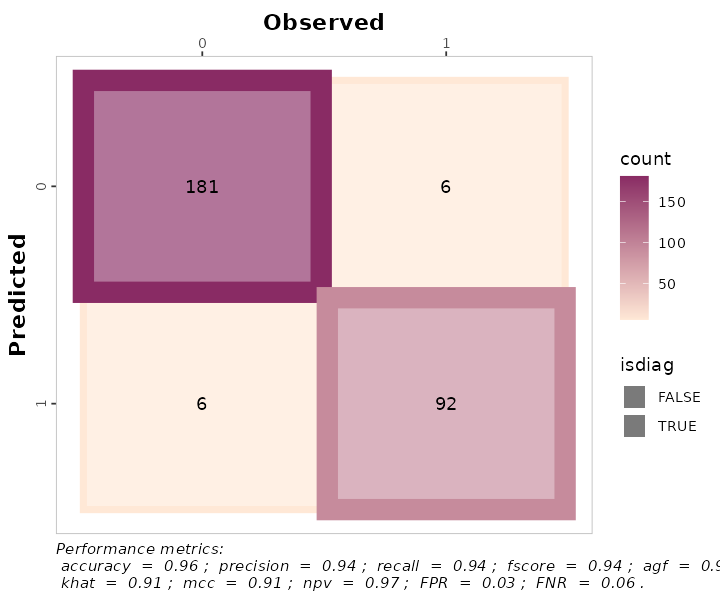multinomial_matrix_metrics <-
maize_phenology %>%
confusion_matrix(obs = actual, pred = predicted,
plot = TRUE,
colors = c(low="grey85" , high="steelblue"),
unit = "count",
# Print metrics_summary
print_metrics = TRUE,
# List of performance metrics
metrics_list = selected_metrics,
# Position (bottom or top)
position_metrics = "bottom")
#> Warning in metrica::fscore(data = ~data, obs = ~actual, pred = ~predicted, :
#> For multiclass cases, the fscore should be estimated at a class level. Please,
#> consider using atom = TRUE
#> Warning in metrica::agf(data = ~data, obs = ~actual, pred = ~predicted, : For
#> multiclass cases, the agf should be estimated at a class level. Please,
#> consider using atom = TRUE
#> Warning in metrica::fmi(data = ~data, obs = ~actual, pred = ~predicted, : The
#> Fowlkes-Mallows Index is not available for multiclass cases. The result has
#> been recorded as NaN.
#> Warning in metrica::preval(data = ~data, obs = ~actual, pred = ~predicted, :
#> For multiclass cases, prevalence should be estimated at a class level. A NaN
#> has been recorded as the result. Please, use atom = TRUE
#> Warning in metrica::preval_t(data = ~data, obs = ~actual, pred = ~predicted, : For multiclass cases, prevalence threshold should be estimated at a class level.
#>       A NaN has been recorded as the result. Please, use atom = TRUE.

multinomial_matrix_metrics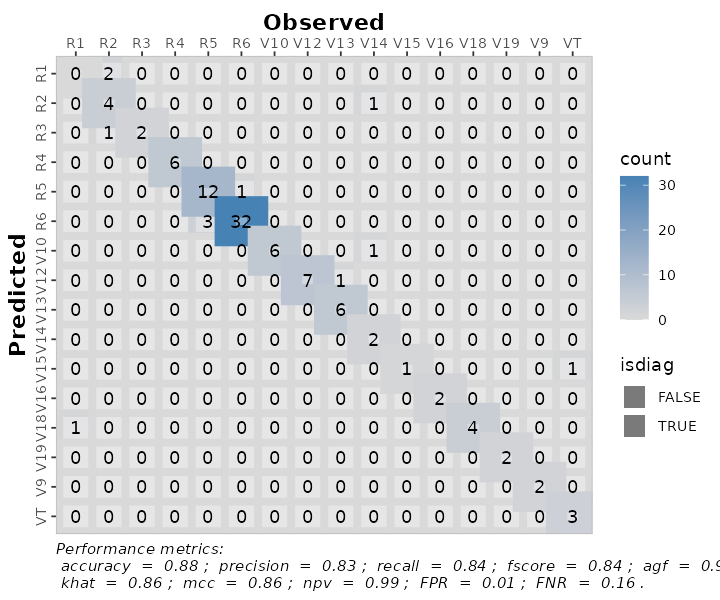Also, as a ggplot element, outputs are flexible of further edition:


binary_matrix_metrics +
# Modify labels
ggplot2::labs(x = "Observed Vegetation",
y = "Predicted Vegetation",
title = "Binary Confusion Matrix")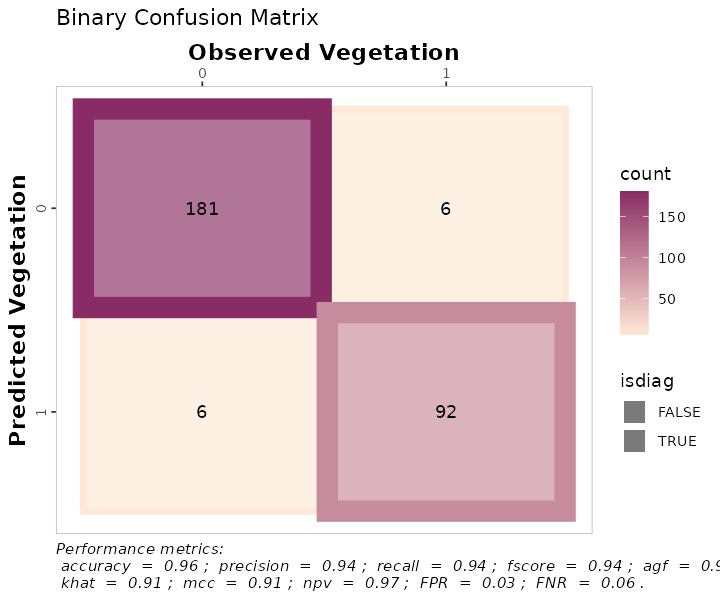multinomial_matrix_metrics +
# Modify labels
ggplot2::labs(x = "Observed Corn Phenology",
y = "Predicted Corn Phenology",
title = "Multinomial Confusion Matrix")+
# Modify theme
ggplot2::theme_light()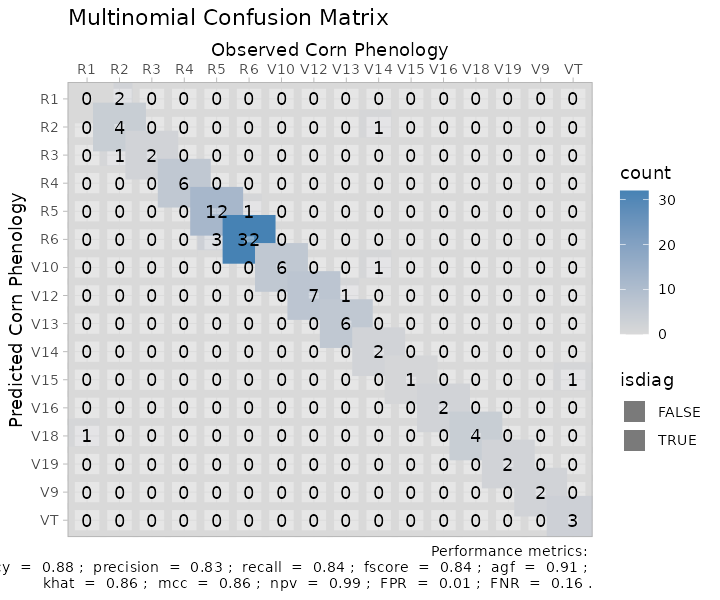## 6. Exporting

To export the metrics summary table, the user can simply write it to file with the function write.csv():

metrics_summary(data = binary_landCover,
obs = obs,
pred = pred,
type = "classification") %>%
write.csv("binary_landcover_metrics_summary.csv")

Similarly, to export a plot, the user can simply write it to file with the function ggsave():


ggsave(plot = multinomial_matrix_metrics,
"multinomial_matrix_metrics.png",
width = 8,
height = 7)